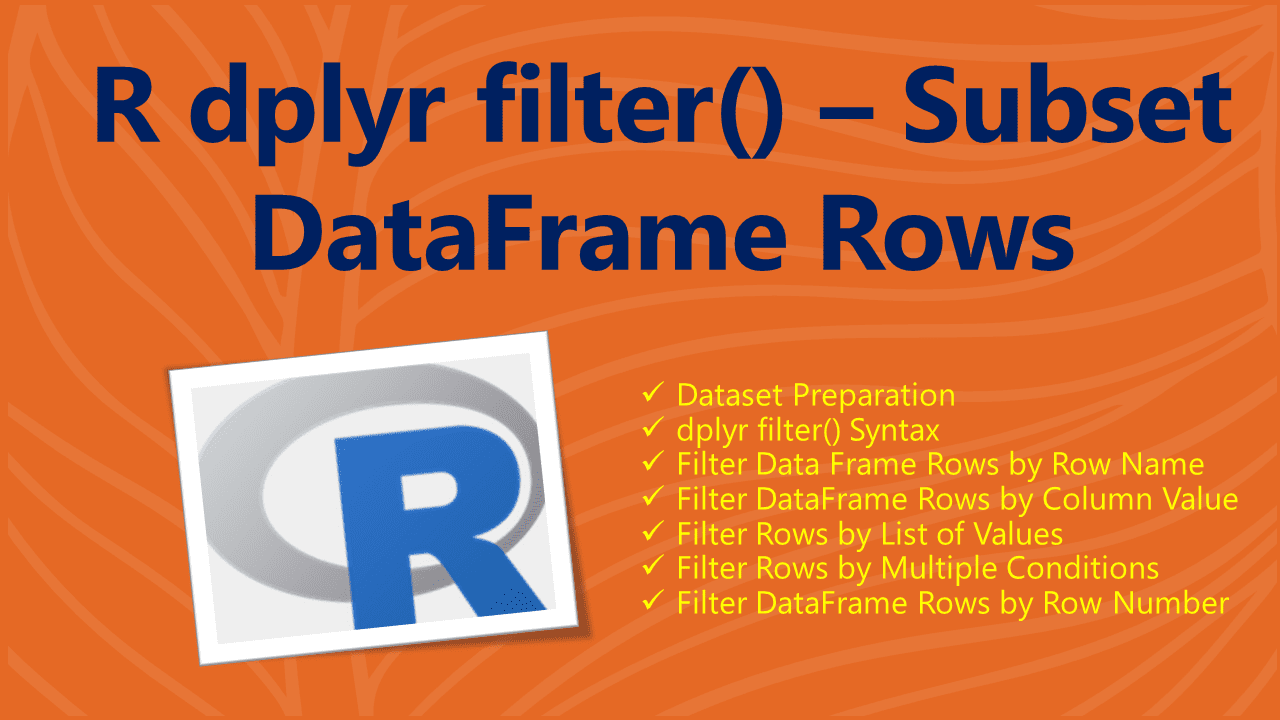# R dplyr filter() – Subset DataFrame Rows

The `filter()` function from `dplyr` package is used to filter the data frame rows in R. Note that filter() doesn’t actually filter the data instead it retains all rows that satisfy the specified condition.

dplyr is an R package that provides a grammar of data manipulation and provides a most used set of verbs that helps data science analysts to solve the most common data manipulation. In order to use this, you have to install it first using `install.packages('dplyr')` and load it using `library(dplyr)`.

## 1. Dataset Preparation

Let’s create an R DataFrame, run these examples and explore the output. If you already have data in CSV you can easily import CSV file to R DataFrame. Also, refer to Import Excel File into R.

``````
# Create DataFrame
df <- data.frame(
id = c(10,11,12,13,14,15,16,17),
name = c('sai','ram','deepika','sahithi','kumar','scott','Don','Lin'),
gender = c('M','M','F','F','M','M','M','F'),
dob = as.Date(c('1990-10-02','1981-3-24','1987-6-14','1985-8-16',
'1995-03-02','1991-6-21','1986-3-24','1990-8-26')),
state = c('CA','NY',NA,NA,'DC','DW','AZ','PH'),
row.names=c('r1','r2','r3','r4','r5','r6','r7','r8')
)
df
``````

Yields below output.

``````
# Output
id    name gender        dob state
r1 10     sai      M 1990-10-02    CA
r2 11     ram      M 1981-03-24    NY
r3 12 deepika      F 1987-06-14  <NA>
r4 13 sahithi      F 1985-08-16  <NA>
r5 14   kumar      M 1995-03-02    DC
r6 15   scott      M 1991-06-21    DW
r7 16     Don      M 1986-03-24    AZ
r8 17     Lin      F 1990-08-26    PH
``````

## 2. dplyr filter() Syntax

Following is the syntax of the `filter()` function from the `dplyr` package.

``````
# Syntax of filter()
filter(x, condition,...)
``````

Parameters

• `x` – Object you wanted to apply a filter on. In our case, it will be a data frame object.
• `condition` – condition you wanted to apply to filter the df.

## 3. Filter Data Frame Rows by Row Name

If you have row names on the data frame and wanted to filter rows by row name in R data frame, use the below approach. By default row names are the incremental sequence numbers assigned at the time of the creation of the R data frame. R also provides a way to assign custom row names while creating the data frame or setting row names on the existing one by using `rownames()` function. To set the column names use `colnames()` function.

``````
# filter() by row name
library('dplyr')
filter(df, rownames(df) == 'r3')
``````

Yields below output. This example returns a row that matches with row name `'r3'`

``````
# Output
id    name gender        dob state
r3 12 deepika      F 1987-06-14  <NA>
``````

## 5. Filter by Column Value

You can also filter dataframe based on column value by specifying the conditions. In the following examples, I have covered how to filter the data frame based on column value. The following example retains rows that `gender` is equal to `'M'`.

``````
# filter() by column Value
library('dplyr')
filter(df, gender == 'M')
``````

Yields below output.

``````
# Output
id name gender        dob state
r7 16  Don      M 1986-03-24    AZ
r8 17  Lin      F 1990-08-26    PH
``````

## 6. Filter Rows by List of Values

If you wanted to choose the rows that match with the list of values, use %in% operator in the condition. The following example retains all rows where the `state` is in the list of values.

``````
# filter() by list of values
filter(df, state %in% c("CA", "AZ", "PH"))
``````

Yields below output.

``````
# Output
id name gender        dob state
r1 10  sai      M 1990-10-02    CA
r7 16  Don      M 1986-03-24    AZ
r8 17  Lin      F 1990-08-26    PH
``````

## 7. Filter Rows by Multiple Conditions

You can also filter data frame rows by multiple conditions in R, all you need to do is use logical operators between the conditions in the expression.

The expressions include comparison operators (==, >, >= ) , logical operators (&, |, !, xor()) , range operators (between(), near()) as well as NA value check against the column values.

``````
# filter() by multiple conditions
library('dplyr')
filter(df, gender == 'M' & id > 15)
``````

Yields below output.

``````
# Output
id name gender        dob state
r7 16  Don      M 1986-03-24    AZ
``````

## 7. Filter Data Frame Rows by Row Number

In order to filter data frame rows by row number or positions in R, we have to use the `slice()` function. this function takes the data frame object as the first argument and the row number you wanted to filter.

``````
# filter() by row number
library('dplyr')
slice(df, 2)
``````

Yields below output.

``````
# Output
id name gender        dob state
r2 11  ram      M 1981-03-24    NY
``````

## 8. Conclusion

In this article, you have learned the syntax and usage of the R filter() function from dplyr package that is used to filter data frame rows by column value, row name, row number, multiple conditions e.t.c.

## References

### Naveen (NNK)

Naveen (NNK) is a Data Engineer with 20+ years of experience in transforming data into actionable insights. Over the years, He has honed his expertise in designing, implementing, and maintaining data pipelines with frameworks like Apache Spark, PySpark, Pandas, R, Hive and Machine Learning. Naveen journey in the field of data engineering has been a continuous learning, innovation, and a strong commitment to data integrity. In this blog, he shares his experiences with the data as he come across. Follow Naveen @ @ LinkedIn